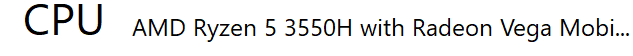# Deep Factorization Machines

Why is my cpu quicker(more examples each second) than your two GPUs?Sorry to miss a number…

Hi @StevenJokes, great question! It is always to point out that CPU may outperform GPU on small networks and less dimension feature inputs.

So you may wonder how to define “a small network”? A simple example: a 100 unit MLP trained on 10 input features may count as a small network. Since there are only 100x10 = 1000 parameters that needs to trained in this network. However, if the input feature dimension increases to 1 million, then the neural net may need more compute resources provided by GPU.

Sorry to miss a number…
GPU is quicker.

I found a question. When I initialized parameters in your way, I could get a similar result as yours. But, if
I done nothing for neural network’ s parameters, the acc is much higher. And for binary classification
question，shouldn’ t its acc exceed 0.5 at least?

I don’t know how doing nothing influences the parameters.
Are all the parameters 0 at start?

OK! I found it!

MXNet will use the default random initialization method: weight parameters for each element are randomly sampled from -0.07 to 0.07 evenly distributed, and all deviation parameters are cleared to zero.
This isn’t binary classification question.please ,I need this code in TensorFlow

I’m just curious…
Test accuracy below 0.5 means… it is worse than just flip the coin. So is this accuracy worth it??

Why use num_inputs = int(sum(field_dims)) as embedding input?
I think the right way should be this: use each dim in field_dims to embedding input feature, and then use each of the embedded vector to do the sum_of_square and square_of_sum(for FM layer) and feed to the NN(NN layer).

I think the code has bug. The correct way is to embedding each of the input sparse features, see my comment below.